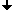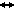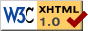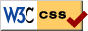Nonlinear Dynamics at the Free University Berlin

Summer 2016

# Seminar: Delay Equations

## Schedule, Summer 2016

Seminar:
Thursday 10.00-12.00, Seminarraum 130, Arnimallee 3

## Description

In this seminar, we want to learn about the theory of delay equations and apply the theory to some examples such as models in control theory, epidemics, or the growth of a sunflower.

## Topics

### Introduction to delay equations 1 - Linear equations, April 21, 2016

• Existence/uniqueness
• Characteristic equation
• Laplace transform
• Fundamental solution
• Maybe: variation of constants, if time allows
• References: , 

### Introduction to delay equations 2 - General theory, April 28, 2016

• Existence/uniqueness
• Continuous dependence
• Continuation of solutions
• Differentiability of solutions
• References: , 

### Introduction to delay equations 3 - Small solutions, May 12, 2016

• The solution map
• Small solutions
• If time allows: compactness
• References: , 

### The zero-number - A Lyapunov function for scalar delay-differential equations, May 19, 2016

• A dropping lemma for delay equations
• Theorem A of , statement and proof
• If time allows: talk shortly about consequences like Morse decomposition
• References: 

### The delayed Mathieu equation, May 26, 2016

• Problem formulation
• Hill's infinite matrix
• Calculation of the stability boundaries
• References: , 

### The global attractor of the sunflower equation, June 2, 2016

• Short introduction to the model equation 
• Characterization of the global attractor 
• References: , , 

### Periodic solutions of a delay equation in epidemics, June 9, 2016

• Explain the model equation
• State and prove Theorem 1 
• Discuss shortly the biological consequences
• References: , 

### Stability of a nuclear reactor with delay, June 23, 2016

• Introduce the model
• Characteristic equations
• Stability in the small region, stability in the large only if time permits
• References: , 

### Pyragas control: Delay stabilization of periodic orbits, June 30, 2016

• Explain Pyragas control
• Show that stabilization near Hopf bifurcation is successful
• References: , , , 

### Pyragas control: The odd-number limitation, July 7, 2016

• Statements of the two theorems and proofs
• Compare autonomous and non-autonomous case
• References: , 

### Lyapunov matrices for time-delay systems

• Lyapunov-Krasovskii functional
• Theorem 4 
• Depending on time: The one-delay case or small examples
• References: , 

## References

•  Kenneth L Cooke and James L Kaplan. A periodicity threshold theorem for epidemics and population growth. Mathematical Biosciences 31(1), pp. 87- 104 (1976).
•  Bernold Fiedler et al. Beyond the odd number limitation of time-delayed feedback control. Handbook of chaos control. John Wiley & Sons, pp. 73-84 (2008).
•  Bernold Fiedler et al. Refuting the odd-number limitation of time-delayed feedback control. Physical Review Letters 98(11), p. 114101 (2007).
•  VD Goryachenko. Stability of a nuclear power generation plant with circulating fuel. Soviet Atomic Energy 21(1), pp. 613-617 (1966).
•  VD Goryachenko and Yu F Trunin. Stability of a nuclear reactor as an object with a time delay. Atomic Energy 23(6), pp. 1265-1269 (1967).
•  Jack K Hale. Theory of functional differential equations. Vol. 3. Springer, 1977.
•  Jack K Hale and Sjoerd M Verduyn Lunel. Introduction to functional differential equations. Vol. 99. Springer, 1993.
•  Edward W Hooton and Andreas Amann. Analytical limitation for time-delayed feedback control in autonomous systems. Physical Review Letters 109(15), p. 154101 (2012).
•  Tamàs Insperger. Stability analysis of periodic delay-differential equations modeling machine tool chatter. PhD dissertation. Budapest University of Technology and Economics, 2002.
•  Tamàs Insperger and Gabor Stépán. Stability chart for the delayed Mathieu equation. Proceedings of the Royal Society of London A: Mathematical, Physical and Engineering Sciences. Vol. 458, pp. 1989-1998 (2002).
•  D Israelsson and A Johnsson. A theory for circumnutations in Helianthus annuus. Physiologia Plantarum 20(4), pp. 957-976 (1967).
•  W Just et al. Beyond the odd number limitation: a bifurcation analysis of time-delayed feedback control. Physical Review E 76(2), p. 026210 (2007).
•  VL Kharitonov and AP Zhabko. Lyapunov-Krasovskii approach to the robust stability analysis of time-delay systems. Automatica 39(1), pp. 15-20 (2003).
•  Vladimir L Kharitonov and E Plischke. Lyapunov matrices for time-delay systems. Systems & Control Letters 55(9), pp. 697-706 (2006).
•  Marcos Lizana. Existence and partial characterization of the global attractor for the sunflower equation. Journal of mathematical analysis and applications 190(1), pp. 1-11 (1995).
•  Marcos Lizana. Global analysis of the sunflower equation with small delay. Nonlinear Analysis: Theory, Methods & Applications 36(6), pp. 697-706 (1999).
•  John Mallet-Paret. Morse decompositions for delay-differential equations. Journal of Differential Equations 72(2), pp. 270-315 (1988).
•  Hiroyuki Nakajima. On analytical properties of delayed feedback control of chaos. In: Physics Letters A 232(3), pp. 207-210 (1997).
•  Kestutis Pyragas. Continuous control of chaos by self-controlling feedback. Physics Letters A 170(6), pp. 421-428 (1992).
•  Hal L Smith. On periodic solutions of a delay integral equation modelling epidemics. Journal of Mathematical Biology 4(1) , pp. 69-80 (1977).Last change: May. 30, 2016
 This page strictly conforms to the XHTML1.0 standard and uses style sheets.